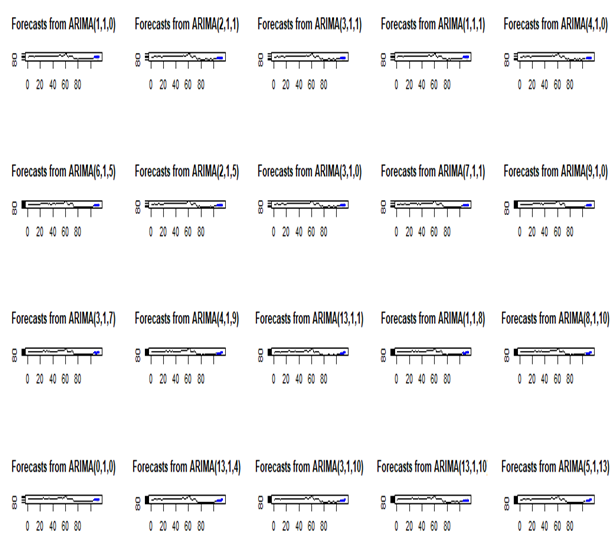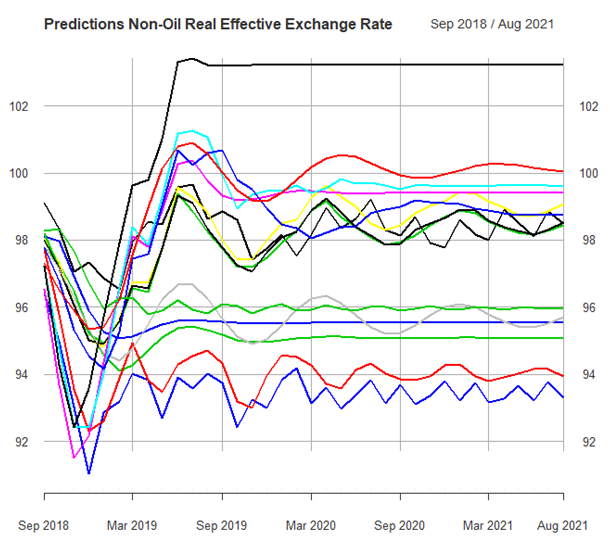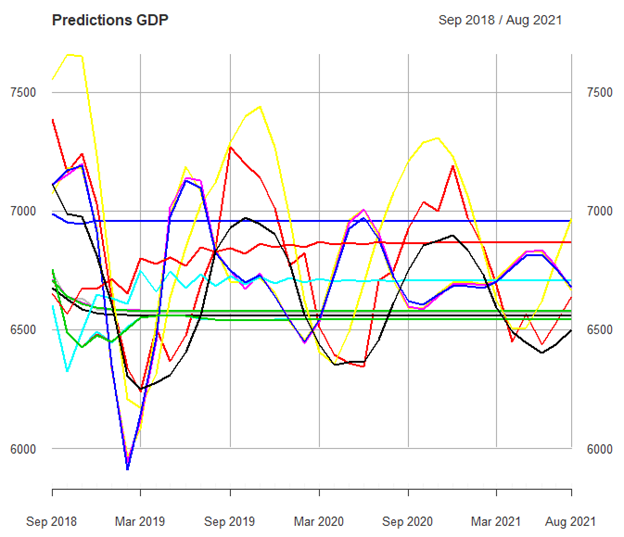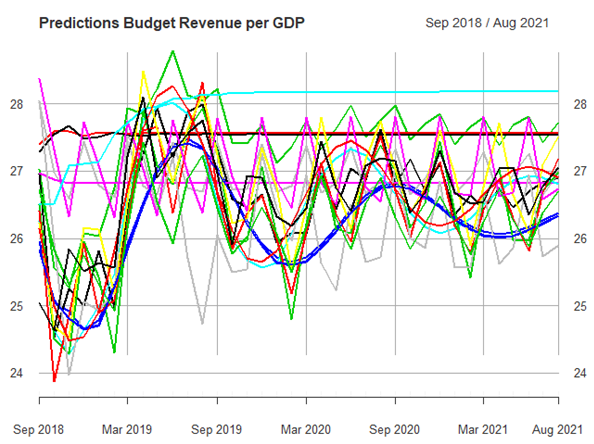It is known that macroeconomic data in Azerbaijan has its own characteristics, reliability being the most important. By that I mean, for example, an unrealistic CPI or unemployment rate. Moreover, the shadow economy is estimated to account for more than 50% of the economy, which means that we observe at best 50% of all economic activity. In that context, doing macro econometric analysis becomes a real challenge and mostly ends in vain (either simple time series or VAR-VEC type regressions). In this paper, I try to find a way to understand future conditions of the economy by independently predicting macroeconomic variables. The point is to capture the future space of variables (e.g., GDP) by randomized ARIMA type models. It can also be extended to build stress-testing for company-specific (mainly banks, as stress-testing is requested by regulators semi-annually) internal variables.

I have on hand 20 monthly macroeconomic variables from January 2010 till August 2018. Here I present only one variable to be compact. The process will be repeated almost exactly the same way for other macroeconomic variables. The variable I have chosen to present is Non-oil Real Effective Exchange Rate as it is one of the main drivers and foci of interest in Azerbaijan. Analyses of the other variables are available upon request.

ARIMA(p,d,q) type models are employed to forecast future values of the selected variable.  First, we plot the data and do initial visual diagnostics. That is, for example, variable looks stationary, trend-stationary, has unit root, has break points or dynamic shifts, shows volatility clustering, etc.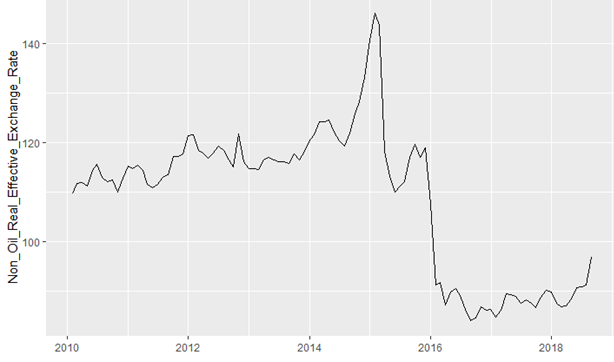Graph 1. Monthly Non-oil Real Effective Exchange Rate (level) (2010-2018)

The pattern does not seem to be a stationary process and we do not see visible seasonality. We cannot detect any deterministic trend. Thus, there is a need to test for the existence of a stochastic trend (unit or more roots, I (d)). Before that we might need to see the log-transformed version of the data as well.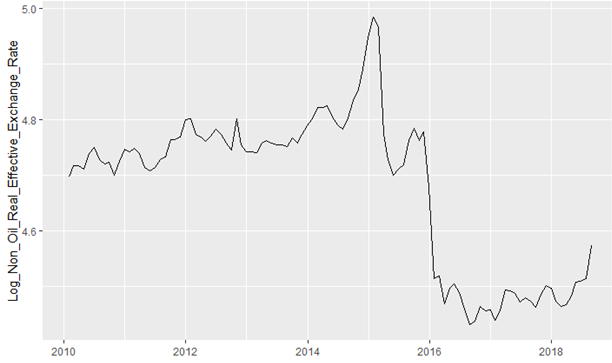Graph 2. Monthly Log-Transformed Non-oil Real Effective Exchange Rate (level)

Log-transformation is known to stabilize data and to help us better analyze data. Moreover, we need to keep in mind that if our variable is unit root process and we have to difference data, the differenced log-transformed data approximates the returns of the variable (log-return). Next, we plot differenced raw and differenced log-transformed data.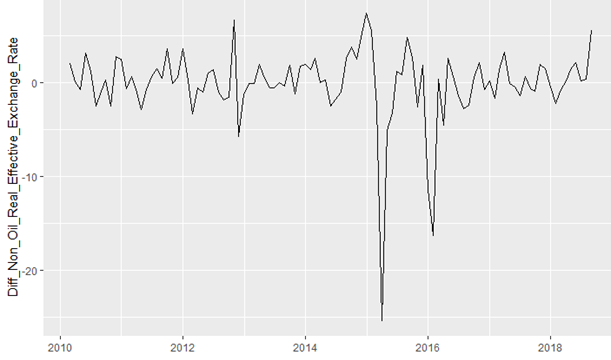Graph 3. First-Differenced Data Non-oil Real Effective Exchange Rate

The differenced data look somewhat stationary. We need to do more rigorous tests to identify the process. As we have a small sample, the power of the tests will be lower than usual. To overcome this, we use three tests and one function. For unit root tests, we use ADF and PP tests. For the stationarity test, we use KPSS. Moreover we use the auto.arima() function of R to understand our data better. Remember, if the data at level is unit root ,I(1), the first-differenced data should be stationary. In general, if data is I(d), d times differenced data should be stationary.

Table 1. Unit Root and Stationarity Tests (p-values)

 LEVEL First-Differenced First-Differenced of Log ADF test 0.68 ADF test <0.01 ADF test <0.01 PP test 0.62 PP test < 0.01 PP test < 0.01 KPSS test <0.01 KPSS test >  0.1 KPSS test >  0.1 Auto Arima ARIMA(2,1,2) Auto Arima ARIMA(2,0,2) Auto Arima ARIMA(1,0,0)

The test results suggest that we should use differenced data because, in the levels, the variable is unit root (I(1)).ADF and PP tests are for unit root testing. Both tests cannot reject the null hypothesis of unit root. The KPSS test is a stationarity test, that is null hypothesis is stationarity, which is rejected at level. Tests for differenced data yields stationary processes. With the help of auto.arima() we end up with two stationary processes. Without any loss of generality, the convention is, to pick a process with fewer parameters. Here, for example, we might choose the AR (1) process over ARMA(2,0,2). Please note that log transformation helps to keep the model simple. In this paper we might exploit both models since we want to end up with many predictions rather than choosing the ‘best’ one.

To see if there is remaining structure in residuals, after fitting the data we need to check Auto-Correlation and Partial Auto-Correlation functions (ACF and PACF).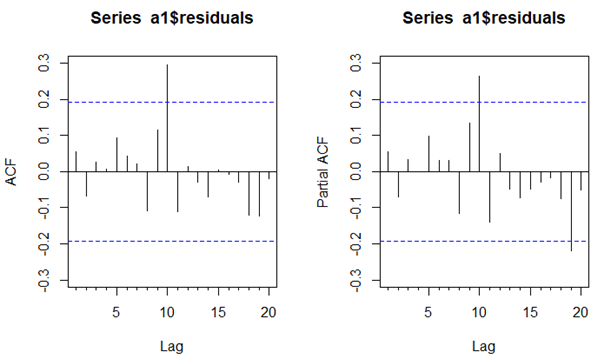Graph 4. Structure in Residuals

It appears to be that there is not much structure left in the residuals as we wanted to end up with. However, there are spikes in the very latter lags of the data, which is a bit suspicious. It might be that the differenced data fits better with the ARMA model. Combined with the graph below, the MA part might be dominating in latter lags.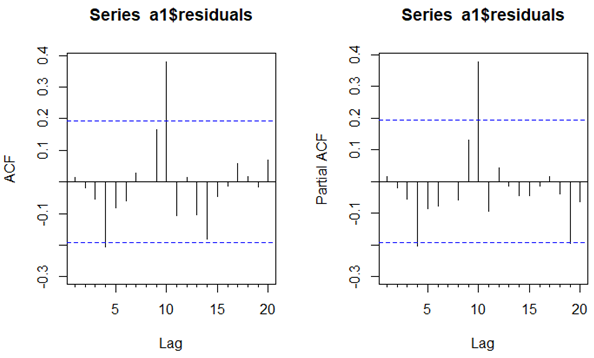Graph 4. Structure in Residuals (log differenced)

Next, we need to check if there are any ARCH effects in the square of the residuals. For that, we need to use some developed tests. We conducted an LM test and a Portmanteau-Q test and the results indicate that there is not enough evidence that the data has ARCH effects (residuals have heteroskedasticicty). Thus, the spikes in residuals are not significant statistically.

We use the forecast() function of R to find possible future values of the variable. However, if we forecast using the ARIMA(1,0,0) model, we will end up with one specific value for each future date. Then, we employ other ARIMA(p,d,q) models to find different future values. We use 20 models by randomized p and q parameters (d is chosen as a result of tests).  As an extra hybrid model, we average forecasts from 20 separate models and end up with 21 models. So, we get, for each month till the end of 2021, 21 predicted future values. Out of them, we sort and choose predicted values as base, worse and the worst.  Based on this, we have stress scenarios. Below, you can see the graphs of forecasts of only one variable (Non-oil Real Effective Exchange Rate) and how we try to capture future possible values. Moreover, as we transformed data and made forecasts on transformed data, we derive back the values to have compatible raw data values.  Note that, we might use the tsclean() function to overcome outlier and gap problems.  We apply all procedures for 20 macroeconomic variables including the presented one. Currently, we are trying to increase the number of different forecasts to have more flexibility. We do not have to limit ourselves to 20 predictions. Theoretical and practical boundaries are still to be researched. Moreover, we can extend our scope to conditional volatility forecasting where possible to gain more predictions and a better picture. As it is randomized, we cannot repeat exactly the same number and model. That gives us freedom to capture real natural randomness. Lastly, we can employ a wider range of ARIMA type models such as ARIMAX, SARIMA, ARFIMA, etc. Moreover, we can employ regularized multinomial logistic regression to have interval predictions and compare them with ARIMA type forecasts.

*Codes and real results are available at request.

Appendix.

Graphs of predictions,20,9,3 – respectively